## 主流开源深度学习框架简介

当下，有许多主流的开源深度学习框架供开发者使用。

主要包括TensorFlow、PyTorch、Keras、Caffe等。

下面是对这几种框架的详细介绍和对比：

## 一、TensorFlow深度学习框架TensorFlow（谷歌出品）：

TensorFlow 是最受欢迎和广泛使用的深度学习框架之一。

TensorFlow使用数据流图来表示计算图，其中节点表示数学操作，边表示数据流动。使用TensorFlow可以利用GPU和分布式计算来加速训练过程。

该框架有着广泛的应用场景，包括图像识别、自然语言处理、语音识别、推荐系统等。同时，TensorFlow也有着丰富的社区支持和文档资源，使其容易学习和使用。

• 适合各种应用，包括计算机视觉、自然语言处理和推荐系统等。
• 提供了丰富的工具和库，用于构建和训练神经网络模型。
• 支持静态计算图和动态计算图。可以在图构建阶段进行优化
• 具有强大的分布式计算能力。
• 支持多种语言接口，包括Python、C++、Java等
• 提供了许多高级操作，如自动微分、数据并行性等
• 易于在各种硬件平台上部署和运行
• 有强大的社区支持和丰富的文档

Tensorflow有三种计算图的构建方式：静态计算图，动态计算图，以及Autograph.
TensorFlow1.0时代，采用的是静态计算图，需要先使用TensorFlow的各种算子创建计算图，再开启一个会话Session，显示执行计算图。

TensorFlow2.0时代，采用的是动态计算图，即每使用一个算子后，该算子会被动态加入到隐含的默认计算图中立即执行得到结果，而无需开启Session。
TensorFlow2.0默认动态计算图（Eager Excution）

如果需要在TensorFlow2.0中使用静态图，可以使用@tf.function装饰器，将普通Python函数转换成对应的TensorFlow计算图构建代码。运行该函数就相当于在TensorFlow1.0中用Session执行代码。
TensorFlow2.0为了确保对老版本tensorflow项目的兼容性，在tf.compat.v1子模块中保留了对TensorFlow1.0静态计算图构建风格的支持。已经不推荐使用了。
tf.Variable在计算图中是一个持续存在的节点,不受作用域的影响,一般的方法是把tf.Variable当做类属性来调用

tf自带数学大小函数

TensorFlow深度学习例子

以下是一个使用TensorFlow保存当前训练模型并在测试集上进行测试的样例代码：
代码中，我们使用了一个简单的线性模型，并在训练过程中将模型保存到了当前目录下的my_model.ckpt文件中。在训练完成后，我们使用测试集进行了模型的测试，输出了测试集上的损失值。

``````import tensorflow as tf
import numpy as np

# 初始化数据和标签
train_data = np.random.rand(1000, 10)
train_labels = np.random.rand(1000, 1)
test_data = np.random.rand(200, 10)
test_labels = np.random.rand(200, 1)

# 创建输入占位符和变量
input_ph = tf.placeholder(tf.float32, shape=[None, 10])
labels_ph = tf.placeholder(tf.float32, shape=[None, 1])
weights = tf.Variable(tf.zeros([10, 1]))
bias = tf.Variable(tf.zeros())

# 创建模型和损失函数
output = tf.matmul(input_ph, weights) + bias
loss = tf.reduce_mean(tf.square(output - labels_ph))

# 创建训练操作和初始化操作
init_op = tf.global_variables_initializer()

# 创建Saver对象
saver = tf.train.Saver()

# 训练模型
with tf.Session() as sess:
sess.run(init_op)
for i in range(100):
_, loss_val = sess.run([train_op, loss], feed_dict={input_ph: train_data, labels_ph: train_labels})
print("Epoch {}: loss = {}".format(i+1, loss_val))

# 保存模型
saver.save(sess, "./my_model.ckpt")

# 在测试集上测试模型
test_loss_val = sess.run(loss, feed_dict={input_ph: test_data, labels_ph: test_labels})
print("Test loss = {}".format(test_loss_val))
``````

```Epoch 1: loss = 0.33758699893951416
Epoch 2: loss = 0.11031775921583176
Epoch 3: loss = 0.09063640236854553
Epoch 4: loss = 0.0888814628124237
Epoch 5: loss = 0.08867537975311279
Epoch 6: loss = 0.08860388398170471
Epoch 7: loss = 0.0885448306798935
Epoch 8: loss = 0.0884876698255539
Epoch 9: loss = 0.0884314551949501
Epoch 10: loss = 0.08837611228227615
Epoch 11: loss = 0.08832161128520966
Epoch 12: loss = 0.08826790004968643
Epoch 13: loss = 0.08821499347686768
Epoch 14: loss = 0.0881628543138504
Epoch 15: loss = 0.08811149001121521
Epoch 16: loss = 0.08806086331605911
Epoch 17: loss = 0.08801095187664032
Epoch 18: loss = 0.08796177059412003
Epoch 19: loss = 0.08791326731443405
Epoch 20: loss = 0.08786546438932419
Epoch 21: loss = 0.08781831711530685
Epoch 22: loss = 0.08777181059122086
Epoch 23: loss = 0.08772596716880798
Epoch 24: loss = 0.08768074214458466
Epoch 25: loss = 0.08763613551855087
Epoch 26: loss = 0.08759212493896484
Epoch 27: loss = 0.08754870295524597
Epoch 28: loss = 0.08750586211681366
Epoch 29: loss = 0.08746359497308731
Epoch 30: loss = 0.08742187172174454
Epoch 31: loss = 0.08738070726394653
Epoch 32: loss = 0.08734006434679031
Epoch 33: loss = 0.08729996532201767
Epoch 34: loss = 0.08726035058498383
Epoch 35: loss = 0.08722126483917236
Epoch 36: loss = 0.0871826633810997
Epoch 37: loss = 0.08714456111192703
Epoch 38: loss = 0.08710692077875137
Epoch 39: loss = 0.08706976473331451
Epoch 40: loss = 0.08703305572271347
Epoch 41: loss = 0.08699680119752884
Epoch 42: loss = 0.08696100115776062
Epoch 43: loss = 0.08692563325166702
Epoch 44: loss = 0.08689068257808685
Epoch 45: loss = 0.0868561640381813
Epoch 46: loss = 0.08682206273078918
Epoch 47: loss = 0.0867883637547493
Epoch 48: loss = 0.08675506711006165
Epoch 49: loss = 0.08672215789556503
Epoch 50: loss = 0.08668963611125946
Epoch 51: loss = 0.08665750920772552
Epoch 52: loss = 0.08662573993206024
Epoch 53: loss = 0.0865943431854248
Epoch 54: loss = 0.08656331896781921
Epoch 55: loss = 0.08653264492750168
Epoch 56: loss = 0.0865023210644722
Epoch 57: loss = 0.08647233247756958
Epoch 58: loss = 0.08644269406795502
Epoch 59: loss = 0.08641338348388672
Epoch 60: loss = 0.08638441562652588
Epoch 61: loss = 0.0863557755947113
Epoch 62: loss = 0.0863274559378624
Epoch 63: loss = 0.08629942685365677
Epoch 64: loss = 0.0862717255949974
Epoch 65: loss = 0.08624432235956192
Epoch 66: loss = 0.0862172394990921
Epoch 67: loss = 0.08619043231010437
Epoch 68: loss = 0.08616391569375992
Epoch 69: loss = 0.08613769710063934
Epoch 70: loss = 0.08611176162958145
Epoch 71: loss = 0.08608610183000565
Epoch 72: loss = 0.08606073260307312
Epoch 73: loss = 0.08603561669588089
Epoch 74: loss = 0.08601076900959015
Epoch 75: loss = 0.08598621189594269
Epoch 76: loss = 0.08596190065145493
Epoch 77: loss = 0.08593783527612686
Epoch 78: loss = 0.08591403067111969
Epoch 79: loss = 0.08589048683643341
Epoch 80: loss = 0.08586718887090683
Epoch 81: loss = 0.08584412187337875
Epoch 82: loss = 0.08582130074501038
Epoch 83: loss = 0.0857987329363823
Epoch 84: loss = 0.08577638119459152
Epoch 85: loss = 0.08575427532196045
Epoch 86: loss = 0.08573239296674728
Epoch 87: loss = 0.08571072667837143
Epoch 88: loss = 0.08568929135799408
Epoch 89: loss = 0.08566807210445404
Epoch 90: loss = 0.08564707636833191
Epoch 91: loss = 0.08562628924846649
Epoch 92: loss = 0.08560571074485779
Epoch 93: loss = 0.0855853483080864
Epoch 94: loss = 0.08556520193815231
Epoch 95: loss = 0.08554524928331375
Epoch 96: loss = 0.0855254977941513
Epoch 97: loss = 0.08550594002008438
Epoch 98: loss = 0.08548659086227417
Epoch 99: loss = 0.08546742796897888
Epoch 100: loss = 0.08544846624135971
Test loss = 0.09260907769203186```

## 二、PyTorch深度学习框架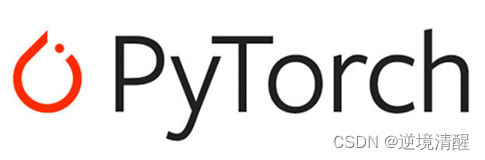PyTorch 是另一个非常受欢迎的深度学习框架。

PyTorch的应用范围非常广泛，包括图像和语音识别、自然语言处理、计算机视觉、推荐系统等领域。

PyTorch具有易于使用、灵活性高和代码可读性好等特点，使得它成为深度学习研究和应用的首选框架之一。

• 易于在GPU上加速训练，具有出色的 GPU 加速性能。
• 提供了广泛的预训练模型和工具包，
• 张量库，用于使用 GPU 和 CPU 进行深度学习。
• 强调动态计算图的构建，可以更灵活地进行模型调整和调试，使得模型构建和调试更加直观。
• 它在灵活性和易用性方面表现出色，特别适合研究和原型开发。
• 具有丰富的工具和库，如Torch方便使用。
• 提供简洁灵活的API，减少代码编写量
• 有活跃的社区和详细的文档支持

PyTorch深度学习例子

#使用 PyTorch 张量将三阶多项式拟合到正弦函数。手动实现转发 并向后通过网络：

``````#使用 PyTorch 张量将三阶多项式拟合到正弦函数。
#手动实现转发 并向后通过网络：

# -*- coding: utf-8 -*-

import torch
import math

dtype = torch.float
device = torch.device("cpu")
# device = torch.device("cuda:0") # Uncomment this to run on GPU

# Create random input and output data
x = torch.linspace(-math.pi, math.pi, 2000, device=device, dtype=dtype)
y = torch.sin(x)

# Randomly initialize weights
a = torch.randn((), device=device, dtype=dtype)
b = torch.randn((), device=device, dtype=dtype)
c = torch.randn((), device=device, dtype=dtype)
d = torch.randn((), device=device, dtype=dtype)

learning_rate = 1e-6
for t in range(2000):
# Forward pass: compute predicted y
y_pred = a + b * x + c * x ** 2 + d * x ** 3

# Compute and print loss
loss = (y_pred - y).pow(2).sum().item()
if t % 100 == 99:
print(t, loss)

# Backprop to compute gradients of a, b, c, d with respect to loss
grad_y_pred = 2.0 * (y_pred - y)

# Update weights using gradient descent

print(f'Result: y = {a.item()} + {b.item()} x + {c.item()} x^2 + {d.item()} x^3')
``````

```99 2351.4306640625
199 1585.7086181640625
299 1071.2376708984375
399 725.2841796875
499 492.4467468261719
599 335.59881591796875
699 229.84210205078125
799 158.46621704101562
899 110.2466812133789
999 77.63826751708984
1099 55.56399154663086
1199 40.60529327392578
1299 30.45751953125
1399 23.5659236907959
1499 18.880510330200195
1599 15.69140911102295
1699 13.518349647521973
1799 12.035942077636719
1899 11.023494720458984
1999 10.331212043762207
Result: y = 0.030692655593156815 + 0.8315182328224182 x + -0.005294993054121733 x^2 + -0.08974269032478333 x^3```

## 三、Keras深度学习框架

Keras（谷歌）：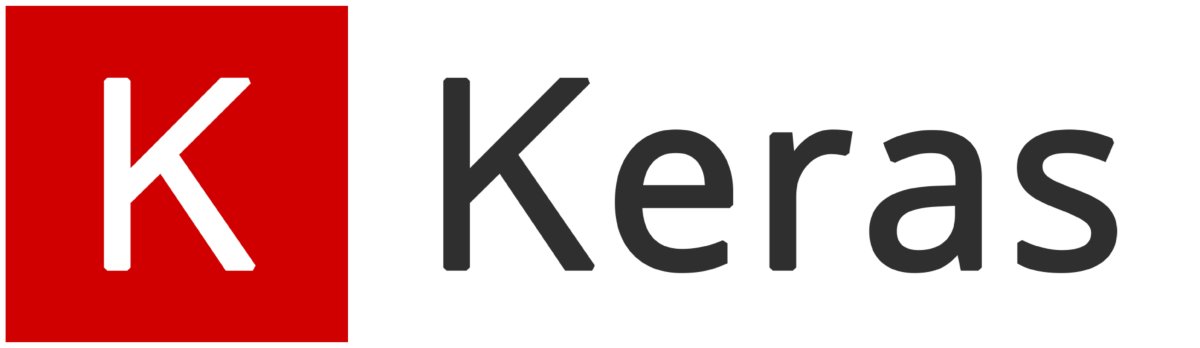Keras（谷歌）（最初由François Chollet开发，现在为TensorFlow官方API）：

Keras 是一个易用且功能强大的，用 Python 编写的高级神经网络 API，它能够以 TensorFlow, CNTK, 或者 Theano 作为后端运行。

Keras 的开发重点是支持快速的实验。能够以最小的时延把你的想法转换为实验结果，是做好研究的关键。

• Keras支持多个后端引擎，可以在 Tensorflow、Theano、CNTK 等框架上运行。
• 提供简洁易用的高级API，尤其适合初学者和快速原型设计
• 具有广泛的模型库、预训练模型和各种工具包，使得模型构建更加高效。
• 可以无缝切换到TensorFlow，以享受其强大的功能和生态系统
• 允许简单而快速的原型设计（由于用户友好，高度模块化，可扩展性）。
• 同时支持卷积神经网络和循环神经网络，以及两者的组合。
• 在 CPU 和 GPU 上无缝运行。

• 用户友好。 Keras 是为人类而不是为机器设计的 API。它把用户体验放在首要和中心位置。Keras 遵循减少认知困难的最佳实践：它提供一致且简单的 API，将常见用例所需的用户操作数量降至最低，并且在用户错误时提供清晰和可操作的反馈。
• 模块化。 模型被理解为由独立的、完全可配置的模块构成的序列或图。这些模块可以以尽可能少的限制组装在一起。特别是神经网络层、损失函数、优化器、初始化方法、激活函数、正则化方法，它们都是可以结合起来构建新模型的模块。
• 易扩展性。 新的模块是很容易添加的（作为新的类和函数），现有的模块已经提供了充足的示例。由于能够轻松地创建可以提高表现力的新模块，Keras 更加适合高级研究。
• 基于 Python 实现。 Keras 没有特定格式的单独配置文件。模型定义在 Python 代码中，这些代码紧凑，易于调试，并且易于扩展。

## 四、Caffe深度学习框架

Caffe（伯克利）Caffe的全称是Convolutional Architecture for Fast Feature Embedding，意为“用于特征提取的卷积架构”，它是一个清晰、高效的深度学习框架，核心语言是C++。

Caffe是一种流行的深度学习框架，是由加州大学伯克利分校的研究人员开发的，用于卷积神经网络（CNN）和其他深度学习模型的训练和部署。

Caffe的主要优点是速度快、易于使用和高度可移植性。

它已被广泛应用于计算机视觉、自然语言处理和语音识别等领域。

Caffe还具有一个强大的社区，提供了许多预训练的模型和可视化工具，使用户可以轻松地构建自己的深度学习模型。

• Caffe 是一个基于 C++ 的深度学习框架，旨在高效地进行卷积运算。
• 它特别适合计算机视觉任务，并在图像分类和物体检测方面表现出色。
• Caffe 提供了简单的配置文件来定义网络结构和超参数。
• 具有高效的 GPU 加速，适合在大规模数据集上训练模型。

## 五、中国深度学习开源框架状况

• 中国深度学习开源框架市场形成三强格局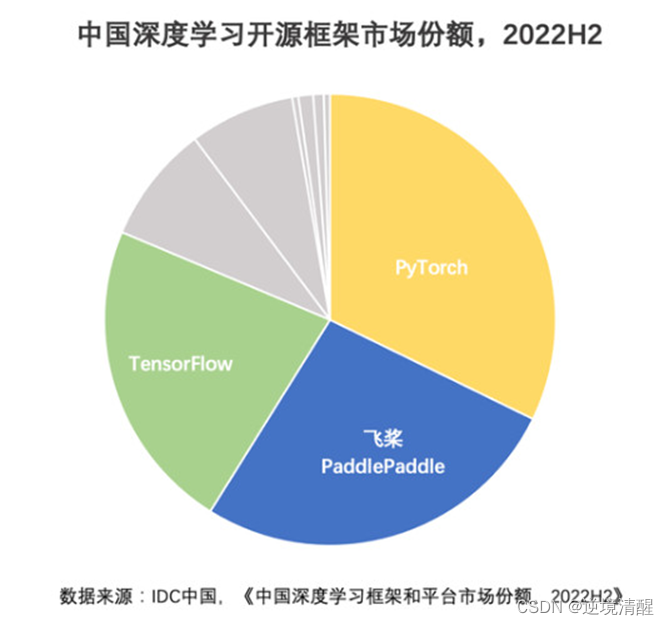国际权威数据调研机构IDC发布《中国深度学习框架和平台市场份额，2022H2》报告。报告显示，百度稳居中国深度学习平台市场综合份额第一，领先优势进一步扩大。中国深度学习开源框架市场形成三强格局，框架市场前三份额超过80%。

## 六、几种框架的对比

目前最受欢迎的深度学习框架包括TensorFlow、PyTorch和Caffe。

据市场研究公司O'Reilly发布的《2019年AI和深度学习市场调查报告》显示，TensorFlow是最受欢迎的深度学习框架，有57.2%的受访者使用它。PyTorch紧随其后，有37.1%的受访者使用它。Caffe和Keras也很受欢迎，分别占据了16.2%和13.7%的市场份额。

 几种常见的深度学习框架在市场上的占比对比（2021） TensorFlow： 超40%， 是目前最流行的深度学习框架之一。 PyTorch： 超25%， 由Facebook开发并维护，近年来逐渐受到关注和广泛应用。 Keras： 超10%， 经常与TensorFlow一起使用，提供了一种更简单易用的框架。 Caffe： 约5%， 市场适用于计算机视觉和图像处理等领域。 MXNet： 约5%， 市场由亚马逊开发并维护，适用于大规模分布式深度学习。

 TensorFlow PyTorch Keras 计算图 静态图 动态图 静态图 语言接口 Python、C++、Java等 Python Python API 丰富 简洁 简洁 硬件支持 广泛 动态图 有限 社区支持 强大 活跃 活跃

 框架 静态图 /动态图 多样化 应用领域 灵活性 与易用性 GPU 加速性能 预训练模型 和工具包 TensorFlow 静态图/动态图 广泛应用 中等 优秀 丰富 PyTorch 动态图 广泛应用 出色 优秀 丰富 Keras 静态图 广泛应用 优秀 出色 丰富 Caffe 静态图 计算机视觉 中等 中等 一般

需要注意的是，这些框架各有优缺点，并且在不同的应用场景下可能有不同的最佳选择。因此，在选择框架时，建议应根据项目需求和研究方向、编程技能和个人喜好来决定，进行评估和比较，最后选择具体的框架。

## 七、其他统计数据

.NET（5+） 用户明年希望使用的前三个选项是 .NET（5+）、.NET MAUI 和 .NET Framework （1.0 - 4.8）。.NET 偏袒性很强 在他们的社区内。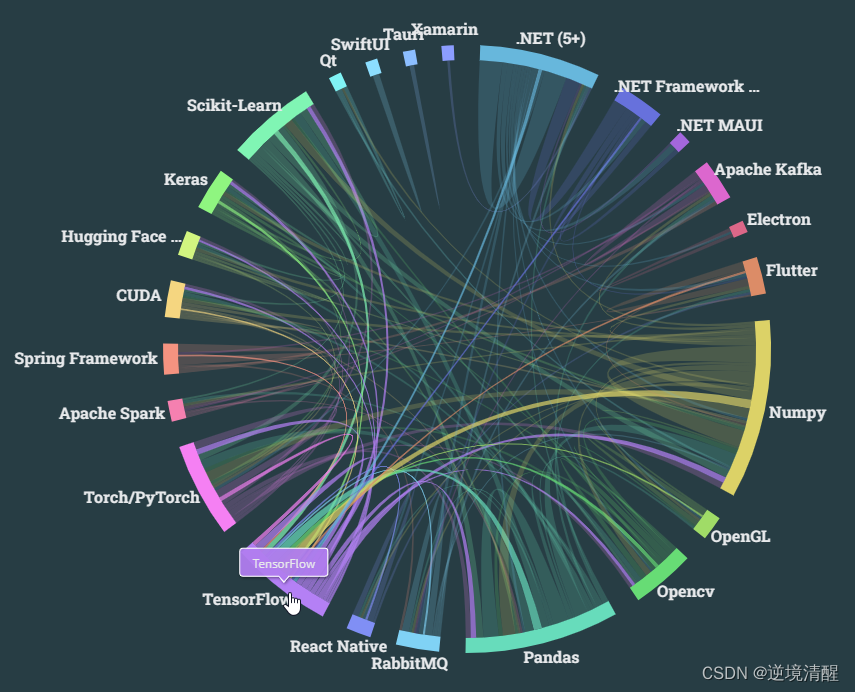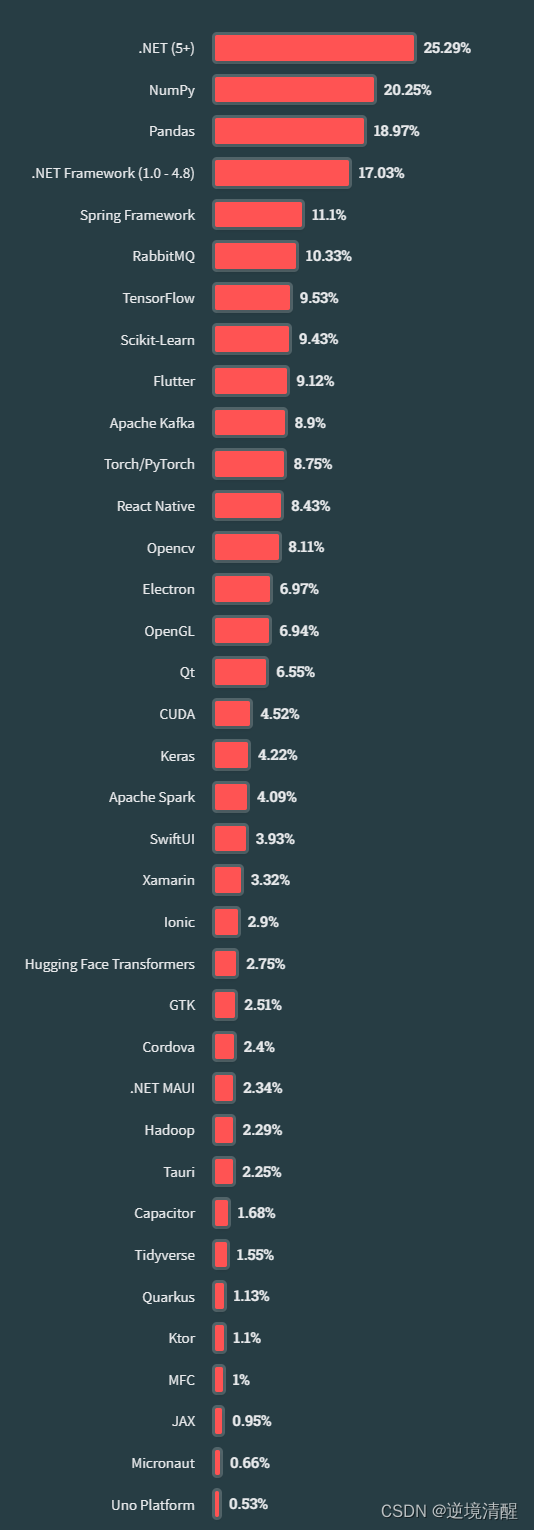推荐阅读：给照片换底色（python+opencv） 猫十二分类 基于大模型的虚拟数字人__虚拟主播实例计算机视觉__基本图像操作（显示、读取、保存） 直方图（颜色直方图、灰度直方图） 直方图均衡化（调节图像亮度、对比度）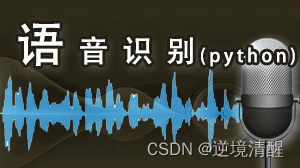Three.js实例详解___旋转的精灵女孩(附完整代码和资源)（一）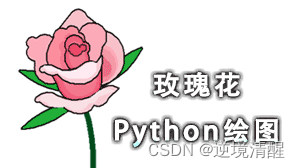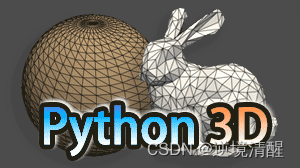立体多层玫瑰绘图源码__玫瑰花python 绘图源码集锦python爱心源代码集锦（18款）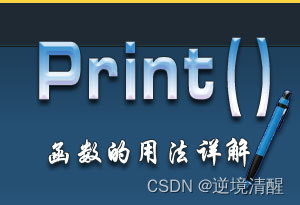用代码过中秋，python海龟月饼你要不要尝一口？草莓熊python turtle绘图（风车版）附源代码皮卡丘python turtle海龟绘图（电力球版）附源代码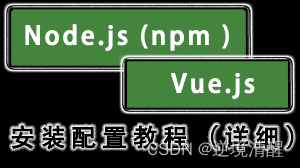Node.js (v19.1.0npm 8.19.3) vue.js安装配置教程（超详细） 色彩颜色对照表（一）(16进制、RGB、CMYK、HSV、中英文名) 2023年4月多家权威机构____编程语言排行榜__薪酬状况​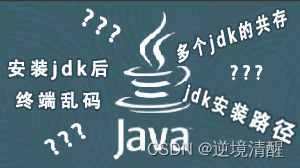【CSDN云IDE】个人使用体验和建议（含超详细操作教程）（python、webGL方向)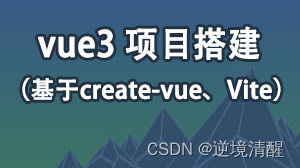vue3 项目搭建教程（基于create-vue，vite，Vite + Vue）2023年春节祝福第二弹——送你一只守护兔，让它温暖每一个你【html5 css3】画会动的小兔子，炫酷充电，字体特 SVG实例详解系列（一）(svg概述、位图和矢量图区别（图解）、SVG应用实例）【程序人生】卡塔尔世界杯元素python海龟绘图（附源代码），世界杯主题前端特效5个（附源码） HTML+CSS+svg绘制精美彩色闪灯圣诞树，HTML+CSS+Js实时新年时间倒数倒计时(附源代码)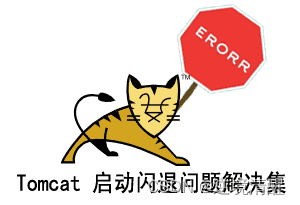tomcat11、tomcat10 安装配置（Windows环境）（详细图文） Tomcat 启动闪退问题解决集（八大类详细）